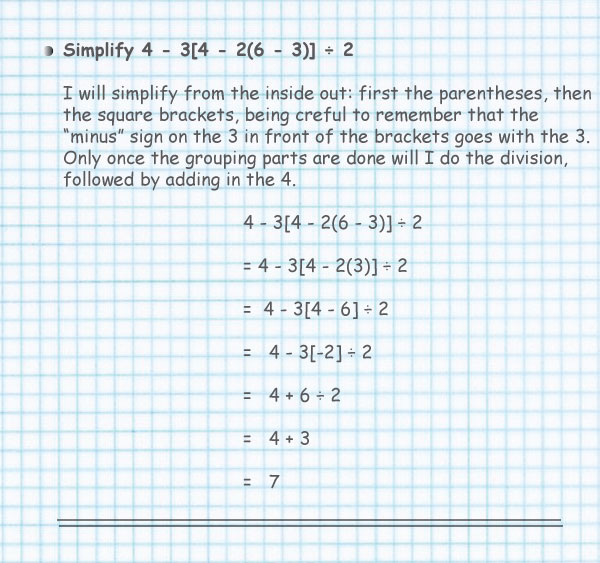BODMAS

"Operations" mean things like add, subtract, multiply, divide, squaring, etc. If it isn't a number it is probably an operation.

Evaluate the following arithmetic expression

## 3 + 4 x 2 ... what part should you calculate first?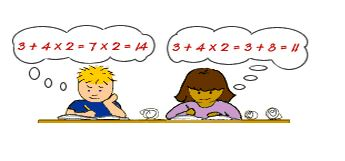Start at the left and go to the right?
Or go from right to left?

Calculate them in the wrong order, and you will get a wrong answer !

 Student 1 Student 2 3 + 4 x 2 3 + 4 x 2 = 7 x 2 = 3 + 8 = 14 = 11

Student 1 performed the operation of addition first, then multiplication

Student 2 performed multiplication first, then addition.

When performing arithmetic operations there can be only one correct answer.

We need a set of rules in order to avoid this kind of confusion.

Mathematicians have devised a standard order of operations for calculations involving more than one arithmetic operation.

 Rule 1 First perform any calculations inside parentheses. Rule 2 Next perform all multiplications and divisions, working from left to right. Rule 3 Lastly, perform all additions and subtractions, working from left to right.

## BODMAS

BODMAS is combined operations of whole numbers and fractions.

The word BODMAS is very important in mathematics especially where more than one operation is involved.

BODMAS gives us the order of operation when any challenge of whole numbers, fractions or decimals involves more than one operation.

We should always remember the word BODMAS which means:

Brackets ( )

Of

Division

Multiplication x

Subtraction -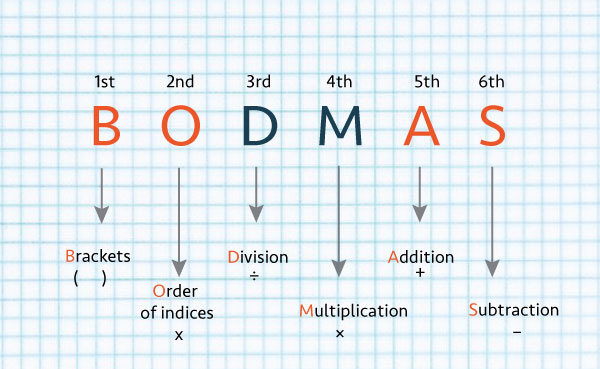Look at the next example.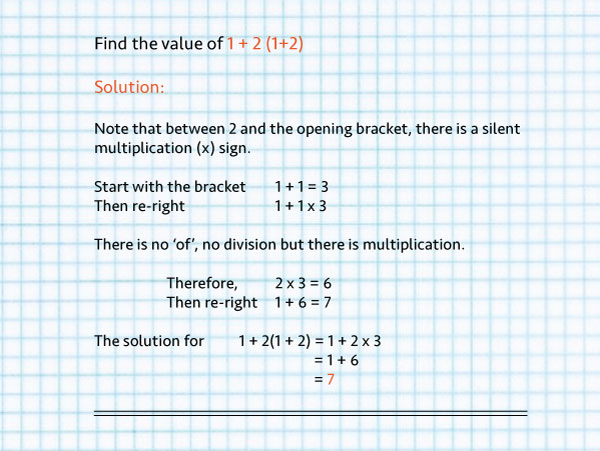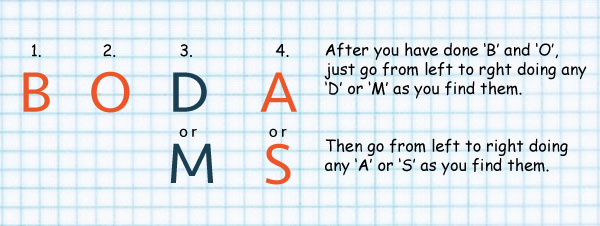## Operations

"Operations" mean things like add, subtract, multiply, divide, squaring, etc. If it isn't a number it is probably an operation.

But, when you see something like...

7 + (6 × 52 + 3)

... what part should you calculate first?

Start at the left and go to the right?
Or go from right to left?

Calculate them in the wrong order, and you will get a wrong answer !

So, long ago people agreed to follow rules when doing calculations, and they are:## How Do I Remember It All ... ? BODMAS !

 B Brackets first O Orders (ie Powers and square roots etc DM Division and Multiplication (left to right) AS Addition and Subtraction (left to right)